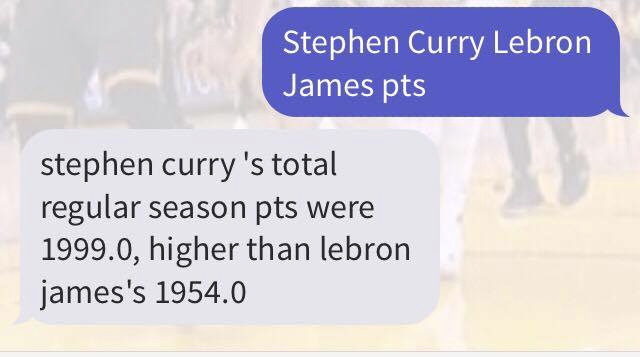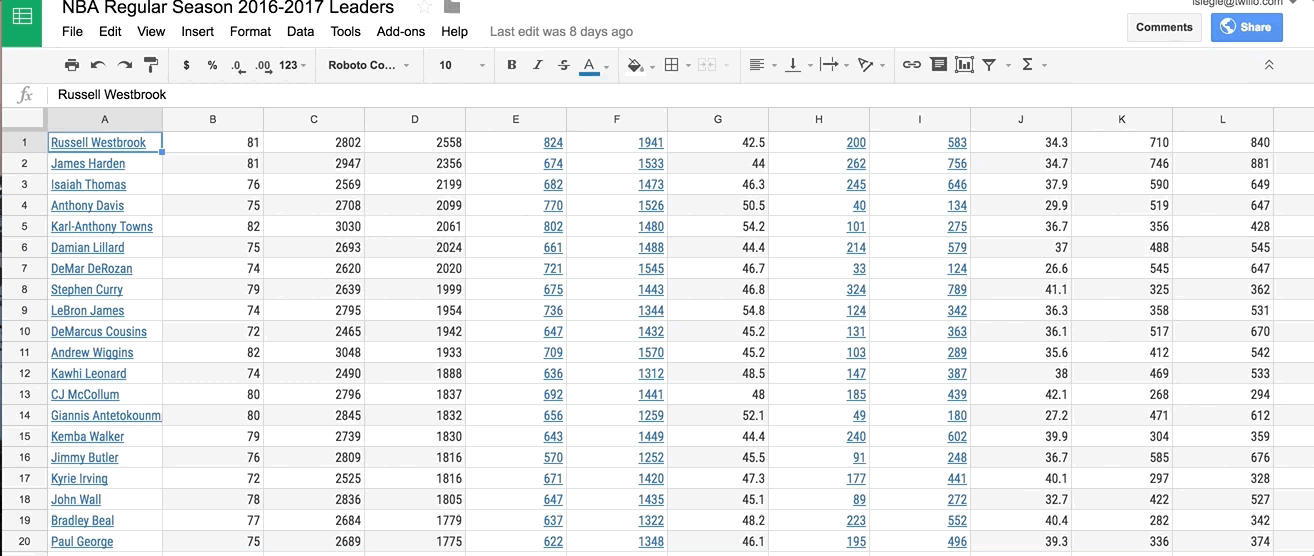Level up your Twilio API skills in TwilioQuest, an educational game for Mac, Windows, and Linux. Download NowData stored in Excel spreadsheets can be hard to read with anything other than Excel and it’s especially tough to compare two specific datasets within all that data. One possible solution is Python. It can do the dirty work of finding the information for us while also being pretty fun.

In this post we will read NBA statistics from a Microsoft Excel sheet using the Openpyxl library. How will we know which statistics to look for and return? Text a Twilio phone number two players and a type of basketball statistic (like total points or three-point-shot-percentage) and then the SMS response will look up the statistics of the corresponding players like so:Want to skip the tutorial and jump right into the code?  No problem, head over to the complete code.

Otherwise, let’s get started.

Getting the Data

This post uses data for each player from the 2016 season on the NBA website here. To get the data I specified which season, season type (playoffs versus regular season), quantifying data type (game average versus total for the season), which dates to look at, and the stats this post uses like age, games played, wins, losses, minutes, points, field goal percentage, and three-point shot percentage, among others. You can see the data in full here, and export it as an Excel file like this.Before we dig into the code and Openpyxl, make sure your Python and Flask development environment is setup.  If you’re new to Python and Flask, this handy guide is a great place to get started. If you’re already familiar with Flask, go ahead and stand up a new empty Flask application.

Your Flask app will need to be visible from the internet so Twilio can send requests to it. Ngrok lets us do this so install it if you haven’t already. Once that’s done, run the following command in your terminal in the directory you’ll put your code in.

```ngrok http 5000
```

This gives us a publicly-accessible URL to the Flask app so we can configure our Twilio phone number.You’ll also need a Twilio phone number to send and receive SMS messages. If you need to get one you can do that here, and let’s make sure it’s configured as shown in this following gif.Parsing Data with Openpyxl

Once you have your environment set up and have acquired a Twilio phone number you can start building the app by installing Openpyxl, an open source Python library that reads and writes Microsoft Excel .xlsx files.

```pip install twilio==6.4.2
pip install openpyxl==2.4.8
```

Open up a new file and save it as main.py. At the very top, include the following imports.

```from flask import Flask, request
from twilio.twiml.messaging_response import MessagingResponse
```

Let’s use two separate lists to store the data we read from the files: one of players and one of their corresponding statistics we want to search (ie. games played, wins, losses, minutes, points, field goal percentage, etc.) These will be used later on to make a dictionary where the keys are the players’ names and the value is their corresponding statistics. Now let’s create a higher-order function called `parse_data_into_dict` in `main.py`, and put in the following code.  This code maps the statistics we’re interested in to different columns of the Excel sheet, represented by letters in `stat_dict`:

```def parse_data_into_dict(data):
list_of_players = []
list_of_stats = []
stat_dict = {
"age":"B", "gp":"C","w":"D","l":"E","min":"F","pts":"G",
"fgm":"H","fga":"I","fg%":"J",
"3pm":"K","3pa":"L","ftm":"M","fta":"N",
"ft%":"O","oreb":"P","dreb":"Q","reb":"R","ast":"S","tov":"T", "stl": "U",
"blk": "V", "pf": "W", "dd2": "X", "td3": "Y"
}
```

Next, let’s fill these lists with the data in the spreadsheet. Start by loading the Excel file using the `load_workbook` function and reading the existing worksheet. Our `parse_data_into_dict` function should now look like this:

```def parse_data_into_dict(data):
list_of_players = []
list_of_stats = []
stat_dict = {
"age":"B", "gp":"C","w":"D","l":"E","min":"F","pts":"G",
"fgm":"H","fga":"I","fg%":"J",
"3pm":"K","3pa":"L","ftm":"M","fta":"N",
"ft%":"O","oreb":"P","dreb":"Q","reb":"R","ast":"S","tov":"T", "stl": "U",
"blk": "V", "pf": "W", "dd2": "X", "td3": "Y"
}
excelfile = 'nbastats.xlsx'
ws = wb[wb.sheetnames]
for row in range(1, ws.max_row+1): #need +1 to get last row!
```

More complex apps and data may have different worksheets within a single workbook. With the data we have, one could be Regular Season while another could be for the Playoffs. We just want the worksheet at index zero since we only have one worksheet,

After we’ve loaded the Excel worksheet, let’s iterate through each cell in the worksheet. Each column of the worksheet is represented by a letter (which is why we made the dictionary above so that the values match the columns in the Excel sheets). Knowing this, let’s add on to our `parse_data_into_dict` function.

```def parse_data_into_dict(data):
list_of_players = []
list_of_stats = []
stat_dict = {
"age":"B", "gp":"C","w":"D","l":"E","min":"F","pts":"G",
"fgm":"H","fga":"I","fg%":"J",
"3pm":"K","3pa":"L","ftm":"M","fta":"N",
"ft%":"O","oreb":"P","dreb":"Q","reb":"R","ast":"S","tov":"T", "stl": "U",
"blk": "V", "pf": "W", "dd2": "X", "td3": "Y"
}
excelfile = 'nba_stats_two_sheets.xlsx'
ws = wb[wb.sheetnames]
for row in range(1, ws.max_row+1): #need +1 to get last row!
for col in "A": #A gets players for texted season
cell_name="{}{}".format(col, row)
list_of_players.append(ws[cell_name].value.lower())
for col in stat_dict[data]: # gets column of whatever statistic
cell_name="{}{}".format(col, row)
#print(ws[cell_name].value)
list_of_stats.append(ws[cell_name].value)
return dict(zip(list_of_players, list_of_stats))
```

The “A” column contains the players’ names so each player in that column is added to the `list_of_players` list. Then, using the `stat_dict` list, we loop through the columns which contain the other stats we are interested in and add those to the separate `list_of_stats` list.

These two lists are then zipped together into one dictionary with players as keys and the corresponding statistic numbers as values. This dictionary will be returned in a send_sms function which we will now make.Building the Flask App and Sending SMS

This send_sms function provides the heart of the code: it’s the route for the Flask app and checks if the body of the incoming SMS is in our dictionary and sends an outbound SMS accordingly.

```app = Flask(__name__)
@app.route('/', methods=['GET', 'POST'])

def send_sms():
msg = request.form['Body'].lower() # convert to lowercase
typomsg = "send 1st + last names of 2 players followed by a stat (GP,W,L,MIN,PTS,FG%,3P%,FT%,REB,AST,STL,BLK). Check for typos!"
player_and_stat = msg.split() #split

if len(player_and_stat) == 5: # check input: 2 players + stat
player1 = player_and_stat + " " + player_and_stat
player2 = player_and_stat + " " + player_and_stat
stat = player_and_stat
player_stat_map = parse_data_into_dict(stat)
if player1 in player_stat_map.keys() and player2 in player_stat_map.keys():
if player_stat_map[player1] > player_stat_map[player2]:
ret = MessagingResponse().message(player1 + " 's total " + str(player_stat_map[player1]) + ", higher than " + player2 + "\'s " + str(player_stat_map[player2]))
else:
ret = MessagingResponse().message(player2 + " 's total " + str(player_stat_map[player2]) + ", higher than " + player1 + "\'s " + str(player_stat_map[player1]))
else: #check
ret = MessagingResponse().message("check both players' names (first and last!)")
else: #idk how many players
ret = MessagingResponse().message(typomsg)
return str(ret)
if __name__ == "__main__":
app.run(debug=True)
```

Let’s break this down. `request.form['Body'].lower()` looks at the input SMS and converts the `Body` to lowercase so it’s easier to compare. Then, we break it up by whitespace and add each piece to a string array. If that array has a length of five (which is what we expect because input should be first and last names of two players followed by a statistic), then we save those two players and the statistic as variables.

Next, we call parse_data_into_dict and use the dictionary it returns to check that it contains the the two players and statistic the message asks for. If it does, we check if the data of one player is greater than the other. Depending on that, a different message is returned. If one or both of the players are not in the dictionary, we return an error message.Run the following command on the command line to run our Flask app.

`python main.py`

Now try out the app.  Text your Twilio number two players (with first and last names) and a statistic like “pts.” It could look something like this.Conclusion

Wow! You just used Openpyxl to read an Excel spreadsheet. What’s next? You can use Openpyxl for financial, baseball, or any sort of data. Here are some more Openpyxl resources and tutorials you may find interesting that go even more in depth.

The completed code is on GitHub. Questions or comments on the data or code used? Find me online here:

Authors
•Lizzie Siegle
Build More With Python
Not ready yet? Talk to an expert.